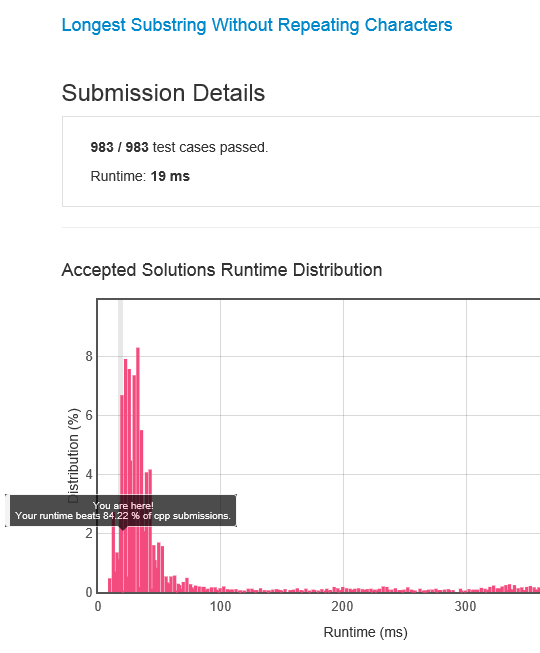# My first Leetcode experience and achievement

By | September 16, 2017

Started to play with Leetcode problems. Pretty addictive activity. The first task I selected was “Longest Substring Without Repeating Characters“. I wrote the code in C# and got a result – 22.9% in “Accepted Solutions Runtime Distribution”. It means that my solution has beaten only one fifth of other submitted implementations. I reprogrammed in C++ and achieved 84%. So it was surprise for me, because I did not expect such difference in my C# and C++ skills.The code:

```class Solution {
public:
int lengthOfLongestSubstring(string s)
{
int len = 0;
int lenstr = s.length();
if (lenstr)
{
const char * substr = s.c_str();
const char * distinct = new char[lenstr+1];
for (int i = 0; i < lenstr; i++)
{
memset((void*)distinct, 0, lenstr + 1);
char * distincttmp = (char*)distinct;
int times = 0;
const char * ch = substr;
for (int k = 0; k < lenstr; k++)
{
const char * pch = strrchr(distinct, *ch);
if (!pch)
{
*distincttmp = *ch;
distincttmp++;
if (times) break;
}
else
times++;
ch++;
}
ch = substr;
char * distincttmp1 = (char*)distinct;
while (*distincttmp1)
{
if (*ch != *distincttmp1)
break;
distincttmp1++;
ch++;
}
int j = ch - substr;
substr++;
if (j == 0) continue;
if ((!len) && (j == distincttmp - distinct))
{
return j;
}
if (j > len)
{
len = j;
}
if ((lenstr - i) <= j)
break;
}
delete[] distinct;
}
return len;
}
}
```